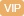| APP | 校园号 | 客服 客服热线：400-863-9889

400-863-9889（2018秋•武城县期末）直接写得数．
42×2= 66÷3= 15×4= 80÷8= 5×40= 100÷5=
4×300= 140÷2= 60×9= 202×6≈ 640÷8= 320÷4=
16×4= 300÷6= 389×2≈
 1 4
+
 2 4
=
 6 7
-
 3 7
=
 5 8
+
 5 8
=
 25 33
-
 14 33
=
 8 25
+
 7 25
=
【考点】整数的乘法及应用整数的除法及应用数的估算分数的加法和减法【专题】计算题．
【分析】根据来的文艺是科技书的4倍，48以25就是艺书的本数，再上8就是图书馆共来书多本．
【解答】解：4×4+48
=24（本）；

【点评】本题的关是求艺书的本数，再据加法的义列出两种书共有的本数．

0/0